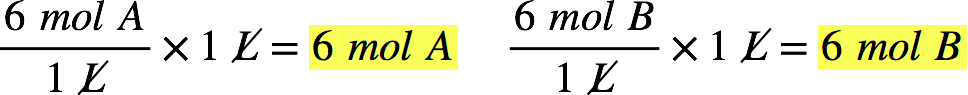# Problem: Consider the reaction:2A(aq) + B(aq) → C(aq)What is the limiting reactant if you mix equal volumes of a 6 M solution of A and a 6 M solution of B?

###### FREE Expert Solution

Recall: Molarity is given by:We first need to calculate the moles of A and B present. Assuming that we have 1 L of each solution, we have:The limiting reactant is the one that produces less amount of product. We need to determine the amount of C formed by A and B.

98% (88 ratings)###### Problem Details

Consider the reaction:

2A(aq) + B(aq) → C(aq)

What is the limiting reactant if you mix equal volumes of a 6 M solution of A and a 6 M solution of B?